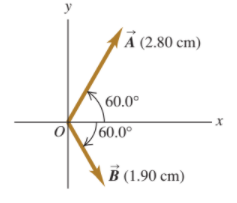×
Get Full Access to University Physics - 13 Edition - Chapter 1 - Problem 39e
Get Full Access to University Physics - 13 Edition - Chapter 1 - Problem 39e

×

# Vector is 2.80 cm long and is 60.0o above the x -axis inISBN: 9780321675460 31

## Solution for problem 39E Chapter 1

University Physics | 13th Edition

• Textbook Solutions
• 2901 Step-by-step solutions solved by professors and subject experts
• Get 24/7 help from StudySoup virtual teaching assistantsUniversity Physics | 13th Edition

4 5 1 334 Reviews
19
2
Problem 39E

Vector $$\overrightarrow{\boldsymbol{A}}$$ is 2.80 cm long and is $$60.0^{\circ}$$ above the $$x$$-axis in the first quadrant. Vector $$\overrightarrow{\boldsymbol{B}}$$ is 1.90 cm long and is $$60.0^{\circ}$$ below the $$x$$-axis in the fourth quadrant (Fig. E1.39). Use components to find the magnitude and direction of (a) $$\overrightarrow{\boldsymbol{A}}+\overrightarrow{\boldsymbol{B}}$$ ; (b) $$\overrightarrow{\boldsymbol{A}}-\overrightarrow{\boldsymbol{B}}$$ ; (c) $$\overrightarrow{\boldsymbol{B}}-\overrightarrow{\boldsymbol{A}}$$ . In each case, sketch the vector addition or subtraction and show that your numerical answers are in qualitative agreement with your sketch.

Figure E1.39Step-by-Step Solution:

Solution 39E Step 1 of 5: A which is 60 from x-axis with magnitude 2.8 cm can be drawn as, 0 Similarly B which is 60 below x-axis with magnitude 1.9 cm can be drawn as,

Step 2 of 5

Step 3 of 5

##### ISBN: 9780321675460

The answer to “Vector is 2.80 cm long and is 60.0o above the x -axis in the first quadrant. Vector is 1.90 cm long and is 60.0o below the x -axis in the fourth quadrant (Fig. E1.35). Use components to find the magnitude and direction of (a) (b) In each case, sketch the vector addition or subtraction and show that your numerical answers are in qualitative agreement with your sketch.” is broken down into a number of easy to follow steps, and 67 words. University Physics was written by and is associated to the ISBN: 9780321675460. This full solution covers the following key subjects: Vector, sketch, quadrant, axis, long. This expansive textbook survival guide covers 26 chapters, and 2929 solutions. The full step-by-step solution to problem: 39E from chapter: 1 was answered by , our top Physics solution expert on 05/06/17, 06:07PM. This textbook survival guide was created for the textbook: University Physics, edition: 13. Since the solution to 39E from 1 chapter was answered, more than 2751 students have viewed the full step-by-step answer.

Unlock Textbook Solution